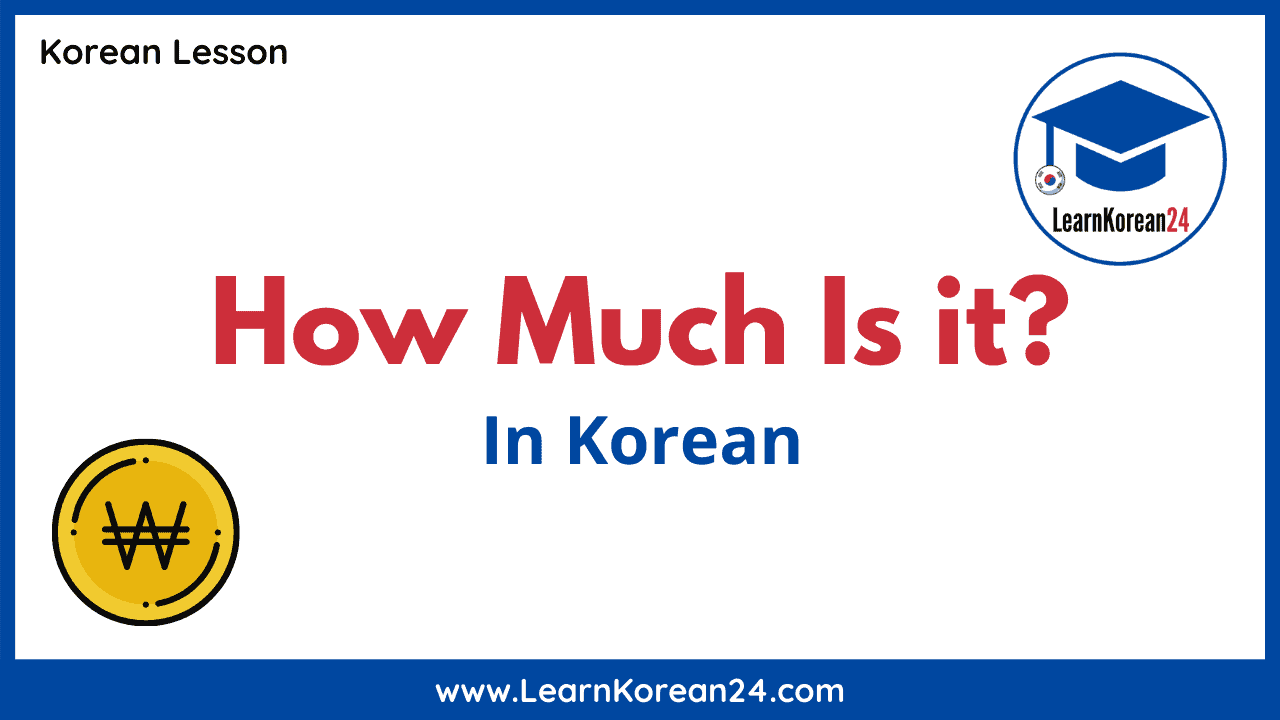In this lesson, you will learn how to ask and answer ‘How much is it?’ in Korean. By learning this expression you will be able to ask the price of things in Korean, which is essential if you ever plan on visiting Korea or purchasing something in Korean won.

Related: How To Count Korean Money

The Korean words in this lesson are written in both Hangul and English. If you’ve not yet learned how to read the Korean alphabet, check out our complete Korean alphabet guide to help you learn how to read in Korean.

## ‘How much is it?’ In Korean

If you can remember how to say ‘What is it?’ from the previous lesson, then it is really easy to learn how to say ‘How much is it?’ in Korean. To recap, ‘What is it?’ is 뭐예요? [mwo-ye-yo?] and this expression is made up of [mwo], meaning ‘what’ and the ending ~예요 [ye-yo], which means ‘is equals to’. To say ‘How much is it?’ in Korean, we simply replace [mwo] with 얼마 [eol-ma] which means ‘how much’.

### 얼마예요? [eol-ma-ye-yo?]

‘How much is it?’ in Korean is 얼마예요? [eol-ma-ye-yo?]. Listen to the audio below to hear how to pronounce this expression.

Let’s look at how to answer the question ‘How much is it?’ in Korean. To say any price in Korean, you first say the number using Sino-Korean numbers, followed by the name of the currency, followed by the ending ~이에요 [i-e-yo], which means ‘is equals to’. Let’s look at an example.

• [cheon] = thousand
• [won] = won (Korean currency)
• 원이에요 [cheon-won-i-e-yo] = It’s one thousand one.

## Example Sentences

Now that you know how to ask and answer ‘How much is it?’ in Korean, you just need to learn Sino-Korean numbers to be able to say any price in Korean. Luckily, Sino-Korean numbers are relatively easy to learn. To learn Korean numbers, check out our complete guide to Sino Korean numbers and Native Korean numbers. Already know Sino-Korean numbers? Then check out the review quiz below to see how well you can answer ‘How much is it?’ in Korean.

## Review Quiz

Check how well you know Sino-Korean numbers and how to answer 얼마예요? with this fun review quiz. Simply look at the price and then choose the correct price in Korean.